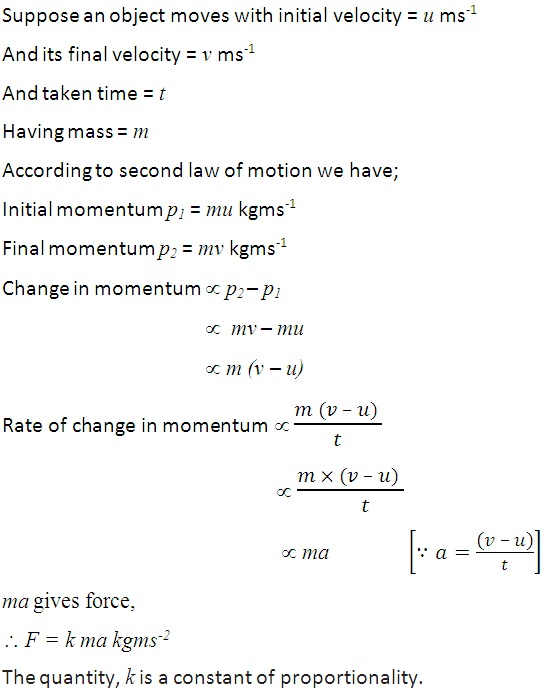Study Materials: CBSE Notes

Our CBSE Notes for Notes 9. Force and Laws of Motion - The Second Law of Motion | Class 9 Science - Toppers Study is the best material for English Medium students cbse board and other state boards students.

Notes ⇒ Class 9th ⇒ Science ⇒ 9. Force and Laws of Motion

# Notes 9. Force and Laws of Motion - The Second Law of Motion | Class 9 Science - Toppers Study

Topper Study classes prepares CBSE Notes on practical base problems and comes out with the best result that helps the students and teachers as well as tutors and so many ecademic coaching classes that they need in practical life. Our CBSE Notes for Notes 9. Force and Laws of Motion - The Second Law of Motion | Class 9 Science - Toppers Study is the best material for English Medium students cbse board and other state boards students.

## Notes 9. Force and Laws of Motion - The Second Law of Motion | Class 9 Science - Toppers Study

CBSE board students who preparing for class 9 ncert solutions maths and Science solved exercise chapter 9. Force and Laws of Motion available and this helps in upcoming exams 2023-2024.

### You can Find Science solution Class 9 Chapter 9. Force and Laws of Motion

• All Chapter review quick revision notes for chapter 9. Force and Laws of Motion Class 9
• NCERT Solutions And Textual questions Answers Class 9 Science
• Extra NCERT Book questions Answers Class 9 Science
• Importatnt key points with additional Assignment and questions bank solved.

Chapter 9 Science class 9

### The Second Law of Motion class 9 Science Chapter 9. Force and Laws of Motion

• Notes 9. Force And Laws Of Motion - The Second Law Of Motion | Class 9 Science - Toppers Study
• Class 9 Ncert Solutions
• Solution Chapter 9. Force And Laws Of Motion Class 9
• Solutions Class 9
• Chapter 9. Force And Laws Of Motion The Second Law Of Motion Class 9

## Notes 9. Force and Laws of Motion - The Second Law of Motion | Class 9 Science - Toppers Study

Chapter 9. Force and Laws of Motion

(2) The Second Law of Motion:

• The acceleration of an object depends on the force applied to it and how we measure a force.
• The second law of motion give the formulla to measure the applied force on an object.
• If an object is to be accelerated, we know that a greater force is required to give a greater velocity.
• The effects produced by an object is depended on  the mass and the velocity of the object.

Momentum: The momentum is an another type of quantity which is represented by Neuton.

Momentum is defined by the product of mass and velocity of an object.

Defintion:

"Momentum is the product of mass and velocity of an object."

It is denoted by the letter "p". It is a vector quantity. As it has both magnitude and direction. It has same direction as velocity of an object.

S.I unit of momentum is kilogram-meter/second (kgms-1

As we know that the change in velocity is made using applying the unbalanced force on the object, so force can change the momentum of an object.

Two factors which can change the momentum of an object:

(i) Change in mass of an object

(ii) Change in velocity of an object

• the force necessary to change the momentum of an object depends on the time rate at which the momentum is changed.

The Second Law of Motion:

The second law of motion states, "the rate of change of momentum of an object is proportional to the applied unbalanced force in the direction of force."

• The Second law of motion gives a method to measure the force applied on an object.

Mathematically find the force from the second law of motion:• The rate of change in momentum is actualy force.
• In decreasing in the rate of change in momentum is also decrease in amount of force.

What to do to decrease the force or the rate in change of momentum in our daily life?

Uses of the second law of motion in daily life:

(i) A cricket player pulls his hand backward while catching ball:

While catching a fast moving cricket ball, a fielder in the ground gradually pulls his hands backwards with the moving ball. This is why, The fast moving ball has a large amount of momemtum, due to its high velocity. This ball carries greater force, that may cause hand injury. Therefore the fielder pulls his hand backward to increase the time,  Due to incerase in time the rate of change in momentum decreases to zero.

(ii) Using a cushioned bed or on a sand bed in high jump:

In a high jump athletic event, the athletes are made to fall either on a cushioned bed or on a sand bed. This is to increase the time of the athlete’s fall to stop after making the jump. This decreases the rate of change of momentum and hence the force.

Important Study materials for classes 06, 07, 08,09,10, 11 and 12. Like CBSE Notes, Notes for Science, Notes for maths, Notes for Social Science, Notes for Accountancy, Notes for Economics, Notes for political Science, Noes for History, Notes For Bussiness Study, Physical Educations, Sample Papers, Test Papers, Mock Test Papers, Support Materials and Books.Mathematics Class - 11th

NCERT Maths book for CBSE Students.

books

## Study Materials List:

##### Notes ⇒ Class 9th ⇒ Science
1. Matter in Our Surroundings
2. Is Matter around us Pure
3. Atoms and Molecules
4. Structure of The Atom
5. The Fundamental Unit of Life
6. Tissues
7. Diversity in Living Organisms
8. Motion
9. Force and Laws of Motion
10. Gravitation
11. Work and Energy
12. Sound
13. Why Do We Fall ill
14. Natural Resources
15. Improvement in Food Resources

New Books